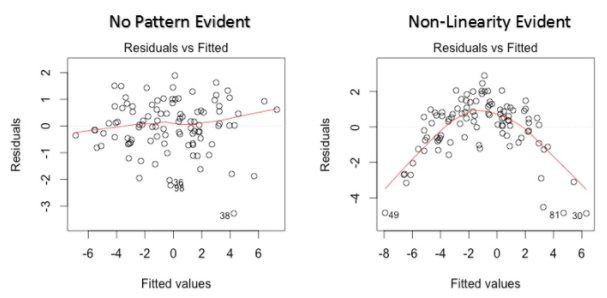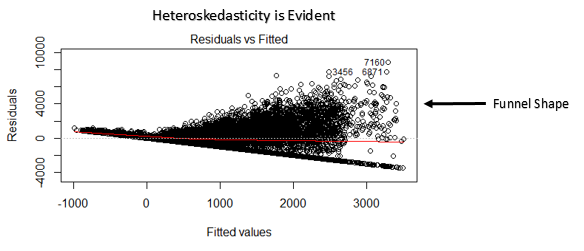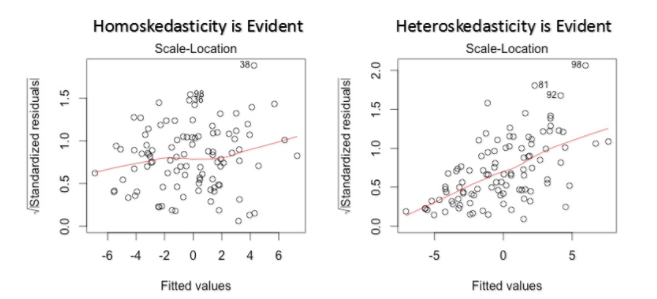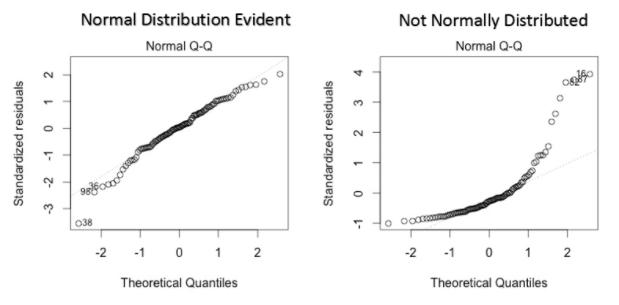# 回归分析的五个基本假设

## 回归分析的五个基本假设

1. 线性性 & 可加性

假设因变量为Y$Y$，自变量为X1$X_1$X2$X_2$，则回归分析的默认假设为Y=b+a1X1+a2X2+ε$Y=b+a_1 X_1+a_2 X_2+\varepsilon$
线性性：X1$X_1$每变动一个单位，Y$Y$相应变动a1$a_1$个单位，与X1$X_1$的绝对数值大小无关。
可加性：X1$X_1$Y$Y$的影响是独立于其他自变量（如X2$X_2$）的。

2. 误差项（ε$\varepsilon$）之间应相互独立。

若不满足这一特性，我们称模型具有自相关性（Autocorrelation）。

3. 自变量（X1X2$X_1，X_2$）之间应相互独立。

若不满足这一特性，我们称模型具有多重共线性性（Multicollinearity）。

4. 误差项（ε$\varepsilon$）的方差应为常数。

若满足这一特性，我们称模型具有同方差性（Homoskedasticity），若不满足，则为异方差性（Heteroskedasticity）。

5. 误差项（ε$\varepsilon$）应呈正态分布。

## 假设失效的影响

1. 线性性 & 可加性

若事实上变量之间的关系不满足线性性（如含有X21$X_1^2$, X31$X_1^3$ 项），或不满足可加性（如含有X1X2$X_1 \cdot X_2$项），则模型将无法很好的描述变量之间的关系，极有可能导致很大的泛化误差（generalization error）

2. 自相关性（Autocorrelation）

自相关性经常发生于时间序列数据集上，后项会受到前项的影响。当自相关性发生的时候，我们测得的标准差往往会偏小，进而会导致置信区间变窄
假设没有自相关性的情况下，自变量X$X$的系数为15.02$15.02$而标准差为2.08$2.08$。假设同一样本是有自相关性的，测得的标准差可能会只有1.20$1.20$，所以置信区间也会从(12.94,17.10)$(12.94,17.10)$缩小到(13.82,16.22)$(13.82,16.22)$

3. 多重共线性性（Multicollinearity）

如果我们发现本应相互独立的自变量们出现了一定程度（甚至高度）的相关性，那我们就很难得知自变量与因变量之间真正的关系了。
当多重共线性性出现的时候，变量之间的联动关系会导致我们测得的标准差偏大，置信区间变宽
采用岭回归，Lasso回归或弹性网（ElasticNet）回归可以一定程度上减少方差，解决多重共线性性问题。因为这些方法，在最小二乘法的基础上，加入了一个与回归系数的模有关的惩罚项，可以收缩模型的系数。
岭回归：=argminβRp(yXβ22+λβ22)$=argmin_{\beta\in\mathbb R^p}(\Vert y-X\beta\Vert_2^2+\lambda\Vert\beta\Vert_2^2)$
Lasso回归：=argminβRp(yXβ22+λβ1)$=argmin_{\beta\in\mathbb R^p}(\Vert y-X\beta\Vert_2^2+\lambda\Vert\beta\Vert_1)$
弹性网回归：=argminβRp(yXβ22+λ1β1+λ2β22)$=argmin_{\beta\in\mathbb R^p}(\Vert y-X\beta\Vert_2^2+\lambda_1\Vert\beta\Vert_1+\lambda_2\Vert\beta\Vert_2^2)$

whereZp=(i=1N|Zi|p)(1/p)

4. 异方差性（Heteroskedasticity）

异方差性的出现意味着误差项的方差不恒定，这常常出现在有异常值（Outlier）的数据集上，如果使用标准的回归模型，这些异常值的重要性往往被高估。在这种情况下，标准差和置信区间不一定会变大还是变小。

5. 误差项（ε$\varepsilon$）应呈正态分布

如果误差项不呈正态分布，意味着置信区间会变得很不稳定，我们往往需要重点关注一些异常的点（误差较大但出现频率较高），来得到更好的模型。

## 假设检验方法

1. 线性性 & 可加性
观察残差（Residual）/估计值（Fitted Value，Y^$\hat{Y}$）图。相较于图一（残差随机分布），图二的残差明显呈现了某种二次型趋势，说明回归模型没有抓住数据的某些非线性特征。
为了克服非线性性的影响，我们可以对自变量做一些非线性变换，如log(X),X,X2..etc$\log(X), \sqrt X, X^2.. etc$

2. 自相关性（Autocorrelation）
观察杜宾-瓦特森统计量（Durbin-Watson Statistic

DW=Tt=2(etet1)2Tt=1e2t

该统计量的值落在(0,4)$(0,4)$内，DW=2$DW=2$意味着没有自相关性，0<DW<2$0表明残差间有正的相关性，2<DW<4$2表明残差间有负的相关性。
经验上，如果DW<1$DW<1$DW>3$DW>3$，则自相关性已经达到了需要示警的水平。如果事先给定了检验的方向（正/负相关性）和置信度α$\alpha$，也可以根据假设检验的思路进行对应计算。

3. 多重共线性性（Multicollinearity）
首先，可以通过观察自变量的散点图（Scatter Plot）来进行初步判断。
然后，针对可能存在多重共线性性的变量，我们观察其方差膨胀系数（VIF–Variance Inflation Factor）
假设回归模型为:

Y=β0+β1X1+β2X2++βkXk+ε

对于变量Xj$X_j$，可证得，其估计系数βj$\beta_j$的方差为：
var^(β^j)=s2(n1)var^(Xj)11R2j

其中唯一与其它自变量有关的值是R2j$R_j^2$R2j$R_j^2$Xj$X_j$关于其它自变量回归的残差：
Xj=β0+β1X1+β2X2++βj1Xj1+βj+1Xj+1++βkXk+ε

11R2j$\frac{1}{1-R_j^2}$便称作VIF$VIF$，若VIF<3$VIF<3$，说明该变量基本不存在多重共线性性问题，若VIF>10$VIF>10$，说明问题比较严重。

4. 异方差性（Heteroskedasticity）
观察残差（Residual）/估计值（Fitted Value，Y^$\hat{Y}$）图。若该图呈现如上图所示的“漏斗形”，即随着Y^$\hat{Y}$的变化，残差有规律的变大或变小，则说明存在明显的异方差性。

或观察残差的标准差（StandardizedResidual$\sqrt{Standardized Residual}$）/估计值图(Scale Location Plot)。也可以看出，异方差数据集呈现出明显的趋势性。

为了克服异方差性的影响，我们可以对因变量做一些非线性变换，如log(Y),Y..etc$\log(Y), \sqrt Y .. etc$

5. 误差项（ε$\varepsilon$）应呈正态分布
方法一：观察Q-Q Plot (quantile-quantile plot)如果误差项满足正态分布，Q-Q Plot里的散点会近似的落在一条直线上。若不满足正态分布，则散点会偏离该直线。

方法二：进行正态检验–如Kolmogorov-Smirnov检验Shapiro–Wilk检验

## 总结

–《归去来兮辞》